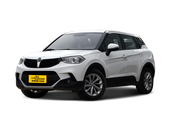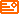• A
• B
• C
• D
• E
• F
• G
• H
• I
• J
• K
• L
• M
• N
• O
• P
• Q
• R
• S
• T
• U
• V
• W
• X
• Y
• Z

• 北京博瑞九龙汽车服务有限公司
• 北京方向华晨汽车销售服务有限公司.
• (未认证) 北京凯瑞翔通汽车销售服务有限公司
• (未认证) 北京祥龙博瑞汽车服务(集团)有限公司三分公司
• (未认证) 北京方向华晨汽车销售服务有限公司
• (未认证) 北京鑫利宝经贸有限公司
• (未认证) 北京东汇盛汽车销售有限公司
• (未认证) 北京三江华晨汽车销售服务有限公司(华晨中华)中华V3

SUV

1.52017款

2016款

•北京博瑞九龙汽车服务有限公司 电话：010-67735393
• 0.00万
• 购车总价：

说明：此结果仅供参考，实际应缴费以当地为准。

询底价
•北京博瑞九龙汽车服务有限公司 电话：010-67735393
• 0.00万
• 购车总价：

说明：此结果仅供参考，实际应缴费以当地为准。

询底价
•北京博瑞九龙汽车服务有限公司 电话：010-67735393
• 0.00万
• 购车总价：

说明：此结果仅供参考，实际应缴费以当地为准。

询底价
•北京博瑞九龙汽车服务有限公司 电话：010-67735393
• 0.00万
• 购车总价：

说明：此结果仅供参考，实际应缴费以当地为准。

询底价
•北京博瑞九龙汽车服务有限公司 电话：010-67735393
• 0.00万
• 购车总价：

说明：此结果仅供参考，实际应缴费以当地为准。

询底价
•北京博瑞九龙汽车服务有限公司 电话：010-67735393
• 0.00万
• 购车总价：

说明：此结果仅供参考，实际应缴费以当地为准。

询底价
•北京博瑞九龙汽车服务有限公司 电话：010-67735393
• 0.00万
• 购车总价：

说明：此结果仅供参考，实际应缴费以当地为准。

询底价
•北京博瑞九龙汽车服务有限公司 电话：010-67735393
• 0.00万
• 购车总价：

说明：此结果仅供参考，实际应缴费以当地为准。

询底价
•北京博瑞九龙汽车服务有限公司 电话：010-67735393
• 0.00万
• 购车总价：

说明：此结果仅供参考，实际应缴费以当地为准。

询底价
•北京博瑞九龙汽车服务有限公司 电话：010-67735393
• 0.00万
• 购车总价：

说明：此结果仅供参考，实际应缴费以当地为准。

询底价
•北京博瑞九龙汽车服务有限公司 电话：010-67735393
• 0.00万
• 购车总价：

说明：此结果仅供参考，实际应缴费以当地为准。

询底价
•北京博瑞九龙汽车服务有限公司 电话：010-67735393
• 0.00万
• 购车总价：

说明：此结果仅供参考，实际应缴费以当地为准。

询底价
•北京博瑞九龙汽车服务有限公司 电话：010-67735393
• 0.00万
• 购车总价：

说明：此结果仅供参考，实际应缴费以当地为准。

询底价
•北京博瑞九龙汽车服务有限公司 电话：010-67735393
• 0.00万
• 购车总价：

说明：此结果仅供参考，实际应缴费以当地为准。

询底价
•北京博瑞九龙汽车服务有限公司 电话：010-67735393
• 0.00万
• 购车总价：

说明：此结果仅供参考，实际应缴费以当地为准。

询底价
•北京博瑞九龙汽车服务有限公司 电话：010-67735393
• 0.00万
• 购车总价：

说明：此结果仅供参考，实际应缴费以当地为准。

询底价
•北京博瑞九龙汽车服务有限公司 电话：010-67735393
• 0.00万
• 购车总价：

说明：此结果仅供参考，实际应缴费以当地为准。

询底价
•北京博瑞九龙汽车服务有限公司 电话：010-67735393
• 0.00万
• 购车总价：

说明：此结果仅供参考，实际应缴费以当地为准。

询底价
•北京博瑞九龙汽车服务有限公司 电话：010-67735393
• 0.00万
• 购车总价：

说明：此结果仅供参考，实际应缴费以当地为准。

询底价
•北京博瑞九龙汽车服务有限公司 电话：010-67735393
• 0.00万
• 购车总价：

说明：此结果仅供参考，实际应缴费以当地为准。

询底价
•北京博瑞九龙汽车服务有限公司 电话：010-67735393
• 0.00万
• 购车总价：

说明：此结果仅供参考，实际应缴费以当地为准。

询底价
•北京博瑞九龙汽车服务有限公司 电话：010-67735393
• 0.00万
• 购车总价：

说明：此结果仅供参考，实际应缴费以当地为准。

询底价
热度排行• 阿斯顿·马丁
• 奥迪
• 阿尔法罗密欧
• ALPINA

• 巴博斯
• 宝骏
• 宝马
• 保时捷
• 北汽制造
• 奔驰
• 奔腾
• 本田
• 比亚迪
• 标致
• 别克
• 宾利
• 布加迪
• 北汽威旺
• 北京
• 北汽绅宝
• 北汽幻速
• 北汽新能源
• 宝沃
• 比速汽车
• 北汽道达

• 昌河
• 长安
• 长城
• 长安商用
• 成功汽车
• 长江EV
• 长安轻车型

• 大众
• 道奇
• 东风
• 东风风神
• 东风小康
• 东南
• DS
• 东风风行
• 东风风度
• 东风风光

• 法拉利
• 菲亚特
• 丰田
• 福迪
• 福特
• 福田汽车
• 福汽启腾
• 风诺

• GMC
• 广汽传祺
• 广汽吉奥
• 观致

• 哈飞
• 海格
• 海马
• 华泰
• 黄海
• 恒天
• 红旗
• 哈弗
• 华颂
• 华凯
• 华泰新能源
• 汉腾汽车

• Jeep
• 江淮
• 江铃
• 捷豹
• 金杯
• 九龙
• 吉利汽车
• 金旅
• 金龙
• 江铃集团轻汽
• 江铃集团新能源
• 君马汽车
• 捷途

• 开瑞
• 凯迪拉克
• 科尼赛克
• 克莱斯勒
• KTM
• 卡威
• 凯翼
• 康迪
• 康迪电动汽车集团

• 猎豹汽车
• 兰博基尼
• 劳斯莱斯
• 雷克萨斯
• 雷诺
• 理念
• 力帆
• 莲花汽车
• 林肯
• 铃木
• 陆风
• 路虎
• 路特斯
• 领志
• 领克
• 零跑汽车

• MG
• MINI
• 马自达
• 玛莎拉蒂
• 迈凯伦
• 摩根

• 纳智捷

• 讴歌
• 欧宝
• 欧朗
• 欧拉
• 欧尚汽车

• 帕加尼

• 奇瑞
• 启辰
• 起亚
• 前途
• 庆铃汽车

• 日产
• 荣威
• 瑞麒汽车
• 如虎
• 瑞驰

• 上汽大通
• smart
• 三菱
• 双环
• 双龙
• 斯巴鲁
• 斯柯达
• 萨博
• 思铭
• 赛麟
• SWM斯威汽车

• TESLA
• 泰卡特
• 腾势

• 威麟
• 威兹曼
• 沃尔沃
• 五菱汽车
• 五十铃
• 潍柴英致
• WEY
• 蔚来
• 威马汽车

• 现代
• 雪佛兰
• 雪铁龙
• 西雅特
• 新特汽车
• 小鹏汽车

• 野马汽车
• 一汽
• 依维柯
• 英菲尼迪
• 永源
• 驭胜

• 中华
• 中兴
• 众泰
• 知豆
• 之诺
• 正道汽车
• A
• B
• C
• D
• E
• F
• G
• H
• I
• J
• K
• L
• M
• N
• O
• P
• Q
• R
• S
• T
• U
• V
• W
• X
• Y
• Z

• 阿斯顿·马丁
• 奥迪
• 阿尔法罗密欧
• ALPINA

• 巴博斯
• 宝骏
• 宝马
• 保时捷
• 北汽制造
• 奔驰
• 奔腾
• 本田
• 比亚迪
• 标致
• 别克
• 宾利
• 布加迪
• 北汽威旺
• 北京
• 北汽绅宝
• 北汽幻速
• 北汽新能源
• 宝沃
• 比速汽车
• 北汽道达

• 昌河
• 长安
• 长城
• 长安商用
• 成功汽车
• 长江EV
• 长安轻车型

• 大众
• 道奇
• 东风
• 东风风神
• 东风小康
• 东南
• DS
• 东风风行
• 东风风度
• 东风风光

• 法拉利
• 菲亚特
• 丰田
• 福迪
• 福特
• 福田汽车
• 福汽启腾
• 风诺

• GMC
• 广汽传祺
• 广汽吉奥
• 观致

• 哈飞
• 海格
• 海马
• 华泰
• 黄海
• 恒天
• 红旗
• 哈弗
• 华颂
• 华凯
• 华泰新能源
• 汉腾汽车

• Jeep
• 江淮
• 江铃
• 捷豹
• 金杯
• 九龙
• 吉利汽车
• 金旅
• 金龙
• 江铃集团轻汽
• 江铃集团新能源
• 君马汽车
• 捷途

• 开瑞
• 凯迪拉克
• 科尼赛克
• 克莱斯勒
• KTM
• 卡威
• 凯翼
• 康迪
• 康迪电动汽车集团

• 猎豹汽车
• 兰博基尼
• 劳斯莱斯
• 雷克萨斯
• 雷诺
• 理念
• 力帆
• 莲花汽车
• 林肯
• 铃木
• 陆风
• 路虎
• 路特斯
• 领志
• 领克
• 零跑汽车

• MG
• MINI
• 马自达
• 玛莎拉蒂
• 迈凯伦
• 摩根

• 纳智捷

• 讴歌
• 欧宝
• 欧朗
• 欧拉
• 欧尚汽车

• 帕加尼

• 奇瑞
• 启辰
• 起亚
• 前途
• 庆铃汽车

• 日产
• 荣威
• 瑞麒汽车
• 如虎
• 瑞驰

• 上汽大通
• smart
• 三菱
• 双环
• 双龙
• 斯巴鲁
• 斯柯达
• 萨博
• 思铭
• 赛麟
• SWM斯威汽车

• TESLA
• 泰卡特
• 腾势

• 威麟
• 威兹曼
• 沃尔沃
• 五菱汽车
• 五十铃
• 潍柴英致
• WEY
• 蔚来
• 威马汽车

• 现代
• 雪佛兰
• 雪铁龙
• 西雅特
• 新特汽车
• 小鹏汽车

• 野马汽车
• 一汽
• 依维柯
• 英菲尼迪
• 永源
• 驭胜

• 中华
• 中兴
• 众泰
• 知豆
• 之诺
• 正道汽车
• A
• B
• C
• D
• E
• F
• G
• H
• I
• J
• K
• L
• M
• N
• O
• P
• Q
• R
• S
• T
• U
• V
• W
• X
• Y
• Z

• 阿斯顿·马丁
• 奥迪
• 阿尔法罗密欧
• ALPINA

• 巴博斯
• 宝骏
• 宝马
• 保时捷
• 北汽制造
• 奔驰
• 奔腾
• 本田
• 比亚迪
• 标致
• 别克
• 宾利
• 布加迪
• 北汽威旺
• 北京
• 北汽绅宝
• 北汽幻速
• 北汽新能源
• 宝沃
• 比速汽车
• 北汽道达

• 昌河
• 长安
• 长城
• 长安商用
• 成功汽车
• 长江EV
• 长安轻车型

• 大众
• 道奇
• 东风
• 东风风神
• 东风小康
• 东南
• DS
• 东风风行
• 东风风度
• 东风风光

• 法拉利
• 菲亚特
• 丰田
• 福迪
• 福特
• 福田汽车
• 福汽启腾
• 风诺

• GMC
• 广汽传祺
• 广汽吉奥
• 观致

• 哈飞
• 海格
• 海马
• 华泰
• 黄海
• 恒天
• 红旗
• 哈弗
• 华颂
• 华凯
• 华泰新能源
• 汉腾汽车

• Jeep
• 江淮
• 江铃
• 捷豹
• 金杯
• 九龙
• 吉利汽车
• 金旅
• 金龙
• 江铃集团轻汽
• 江铃集团新能源
• 君马汽车
• 捷途

• 开瑞
• 凯迪拉克
• 科尼赛克
• 克莱斯勒
• KTM
• 卡威
• 凯翼
• 康迪
• 康迪电动汽车集团

• 猎豹汽车
• 兰博基尼
• 劳斯莱斯
• 雷克萨斯
• 雷诺
• 理念
• 力帆
• 莲花汽车
• 林肯
• 铃木
• 陆风
• 路虎
• 路特斯
• 领志
• 领克
• 零跑汽车

• MG
• MINI
• 马自达
• 玛莎拉蒂
• 迈凯伦
• 摩根

• 纳智捷

• 讴歌
• 欧宝
• 欧朗
• 欧拉
• 欧尚汽车

• 帕加尼

• 奇瑞
• 启辰
• 起亚
• 前途
• 庆铃汽车

• 日产
• 荣威
• 瑞麒汽车
• 如虎
• 瑞驰

• 上汽大通
• smart
• 三菱
• 双环
• 双龙
• 斯巴鲁
• 斯柯达
• 萨博
• 思铭
• 赛麟
• SWM斯威汽车

• TESLA
• 泰卡特
• 腾势

• 威麟
• 威兹曼
• 沃尔沃
• 五菱汽车
• 五十铃
• 潍柴英致
• WEY
• 蔚来
• 威马汽车

• 现代
• 雪佛兰
• 雪铁龙
• 西雅特
• 新特汽车
• 小鹏汽车

• 野马汽车
• 一汽
• 依维柯
• 英菲尼迪
• 永源
• 驭胜

• 中华
• 中兴
• 众泰
• 知豆
• 之诺
• 正道汽车
• A
• B
• C
• D
• E
• F
• G
• H
• I
• J
• K
• L
• M
• N
• O
• P
• Q
• R
• S
• T
• U
• V
• W
• X
• Y
• Z